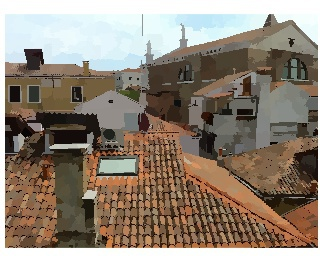Quick shift is a mode seeking algorithm (like mean shift) which instead of iteratively shifting each point towards a local mean instead forms a tree of links to the nearest neighbor which increases the density. For a more in-depth description of the algorithm, see our API reference for quick shift

# Using quick shift to find superpixels

This demo shows quick shift in a simple superpixelization problem where we seek to segment this image:The image we wish to segment

As a feature vector, we choose the LAB colorspace representation of the image augmented with the x,y location of the pixel. vl_quickseg is a convenient wrapper function which takes care of the transformation of the image to LAB and performs segmentation, making our job as easy as:

ratio = 0.5;
kernelsize = 2;
Iseg = vl_quickseg(I, ratio, kernelsize, maxdist);


where ratio is the tradeoff between color importance and spatial importance (larger values give more importance to color), kernelsize is the size of the kernel used to estimate the density, and maxdist is the maximum distance between points in the feature space that may be linked if the density is increased.The effect of maxdist on the superpixelization. As we increase maxdist, superpixels become larger and larger since we can link less similar points. Top: maxdist=10. Bottom: maxdist=20.

# Multiple segmentations

Quick shift arranges all of the data points into a tree where parents in the tree are the nearest neighbors in the feature space which increase the estimate of the density. By imposing a limit on the distance between nearest neighbors (maxdist), we decrease the amount of computation required to search for the nearest neighbors. However, we also break our tree into a forest, because local modes of the density will now have no neighbor which is close enough in the feature space to form a link.

In the previous section, we created a superpixel segmentation by taking each of the trees in this forest as a distinct cluster. However, since maxdist simply prevents new links from forming, the segmentation formed by every dist < maxdist is contained in the result. vl_quickvis lets us visualize this by running quick shift once and forming multiple segmentations by cutting links in the tree which are smaller and smaller.

maxdist = 50;
ndists = 10;
Iedge = vl_quickvis(I, ratio, kernelsize, maxdist, ndists)
imagesc(Iedge);
axis equal off tight;
colormap gray;A visualization of multiple maxdist thresholds on a single image. Here, boundaries are colored by the largest maxdist where the boundary is preserved.